< class="sp-layer sp-black sp-padding" data-position="topCenter" data-horizontal="0" data-vertical="50" data-show-transition="right" data-show-delay="500">< class="sp-layer sp-black sp-padding" data-position="topCenter" data-horizontal="0" data-vertical="50" data-show-transition="right" data-show-delay="500">< class="sp-layer sp-black sp-padding" data-position="topCenter" data-horizontal="0" data-vertical="50" data-show-transition="right" data-show-delay="500">< class="sp-layer sp-black sp-padding" data-position="topCenter" data-horizontal="0" data-vertical="50" data-show-transition="right" data-show-delay="500">< class="sp-layer sp-black sp-padding" data-position="topCenter" data-horizontal="0" data-vertical="50" data-show-transition="right" data-show-delay="500">< class="sp-layer sp-black sp-padding" data-position="topCenter" data-horizontal="0" data-vertical="50" data-show-transition="right" data-show-delay="500">< class="sp-layer sp-black sp-padding" data-position="topCenter" data-horizontal="0" data-vertical="50" data-show-transition="right" data-show-delay="500">< class="sp-layer sp-black sp-padding" data-position="topCenter" data-horizontal="0" data-vertical="50" data-show-transition="right" data-show-delay="500">< class="sp-layer sp-black sp-padding" data-position="topCenter" data-horizontal="0" data-vertical="50" data-show-transition="right" data-show-delay="500">< class="sp-layer sp-black sp-padding" data-position="topCenter" data-horizontal="0" data-vertical="50" data-show-transition="right" data-show-delay="500">< class="sp-layer sp-black sp-padding" data-position="topCenter" data-horizontal="0" data-vertical="50" data-show-transition="right" data-show-delay="500">< class="sp-layer sp-black sp-padding" data-position="topCenter" data-horizontal="0" data-vertical="50" data-show-transition="right" data-show-delay="500">< class="sp-layer sp-black sp-padding" data-position="topCenter" data-horizontal="0" data-vertical="50" data-show-transition="right" data-show-delay="500">< class="sp-layer sp-black sp-padding" data-position="topCenter" data-horizontal="0" data-vertical="50" data-show-transition="right" data-show-delay="500">< class="sp-layer sp-black sp-padding" data-position="topCenter" data-horizontal="0" data-vertical="50" data-show-transition="right" data-show-delay="500">< class="sp-layer sp-black sp-padding" data-position="topCenter" data-horizontal="0" data-vertical="50" data-show-transition="right" data-show-delay="500">< class="sp-layer sp-black sp-padding" data-position="topCenter" data-horizontal="0" data-vertical="50" data-show-transition="right" data-show-delay="500">< class="sp-layer sp-black sp-padding" data-position="topCenter" data-horizontal="0" data-vertical="50" data-show-transition="right" data-show-delay="500">< class="sp-layer sp-black sp-padding" data-position="topCenter" data-horizontal="0" data-vertical="50" data-show-transition="right" data-show-delay="500">< class="sp-layer sp-black sp-padding" data-position="topCenter" data-horizontal="0" data-vertical="50" data-show-transition="right" data-show-delay="500">< class="sp-layer sp-black sp-padding" data-position="topCenter" data-horizontal="0" data-vertical="50" data-show-transition="right" data-show-delay="500">< class="sp-layer sp-black sp-padding" data-position="topCenter" data-horizontal="0" data-vertical="50" data-show-transition="right" data-show-delay="500">< class="sp-layer sp-black sp-padding" data-position="topCenter" data-horizontal="0" data-vertical="50" data-show-transition="right" data-show-delay="500">< class="sp-layer sp-black sp-padding" data-position="topCenter" data-horizontal="0" data-vertical="50" data-show-transition="right" data-show-delay="500">< class="sp-layer sp-black sp-padding" data-position="topCenter" data-horizontal="0" data-vertical="50" data-show-transition="right" data-show-delay="500">< class="sp-layer sp-black sp-padding" data-position="topCenter" data-horizontal="0" data-vertical="50" data-show-transition="right" data-show-delay="500">< class="sp-layer sp-black sp-padding" data-position="topCenter" data-horizontal="0" data-vertical="50" data-show-transition="right" data-show-delay="500">< class="sp-layer sp-black sp-padding" data-position="topCenter" data-horizontal="0" data-vertical="50" data-show-transition="right" data-show-delay="500">< class="sp-layer sp-black sp-padding" data-position="topCenter" data-horizontal="0" data-vertical="50" data-show-transition="right" data-show-delay="500">< class="sp-layer sp-black sp-padding" data-position="topCenter" data-horizontal="0" data-vertical="50" data-show-transition="right" data-show-delay="500">< class="sp-layer sp-black sp-padding" data-position="topCenter" data-horizontal="0" data-vertical="50" data-show-transition="right" data-show-delay="500">< class="sp-layer sp-black sp-padding" data-position="topCenter" data-horizontal="0" data-vertical="50" data-show-transition="right" data-show-delay="500">< class="sp-layer sp-black sp-padding" data-position="topCenter" data-horizontal="0" data-vertical="50" data-show-transition="right" data-show-delay="500">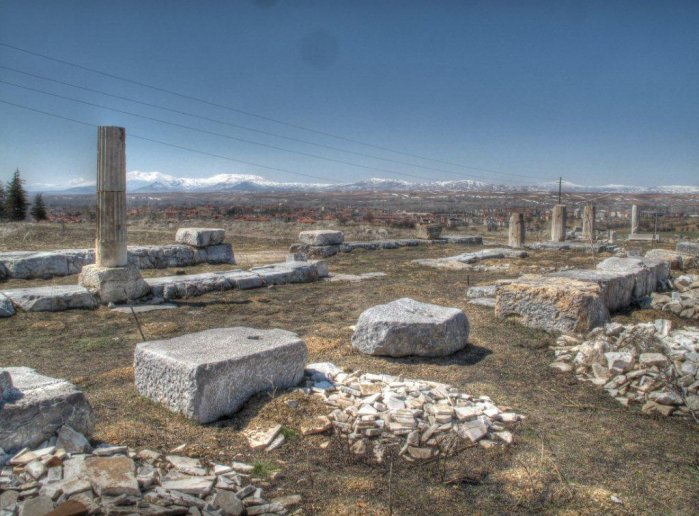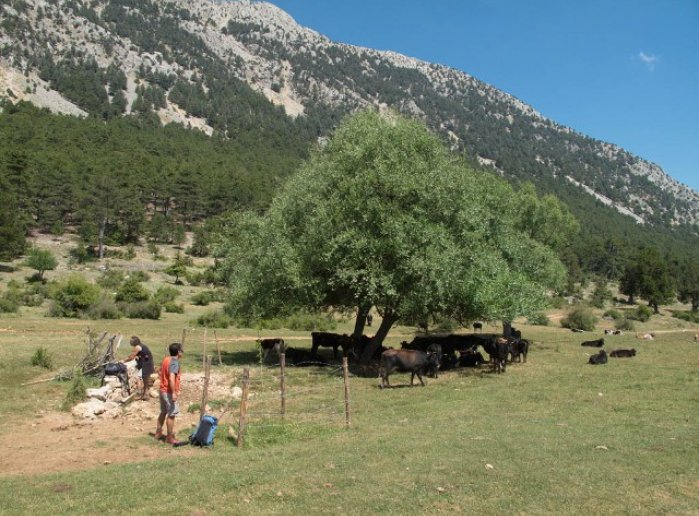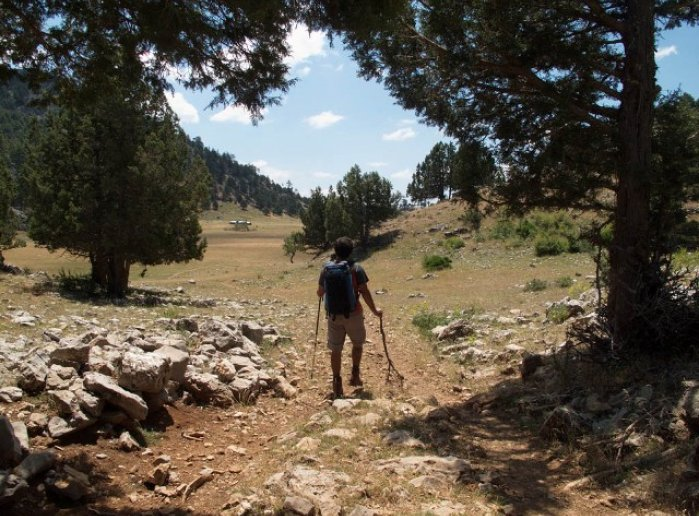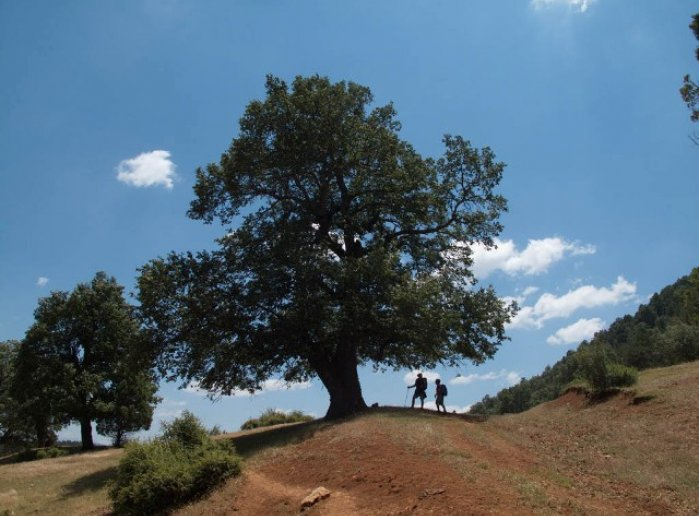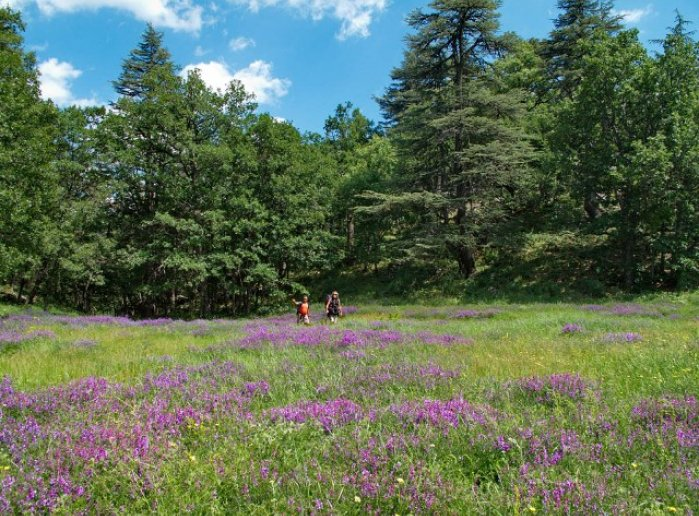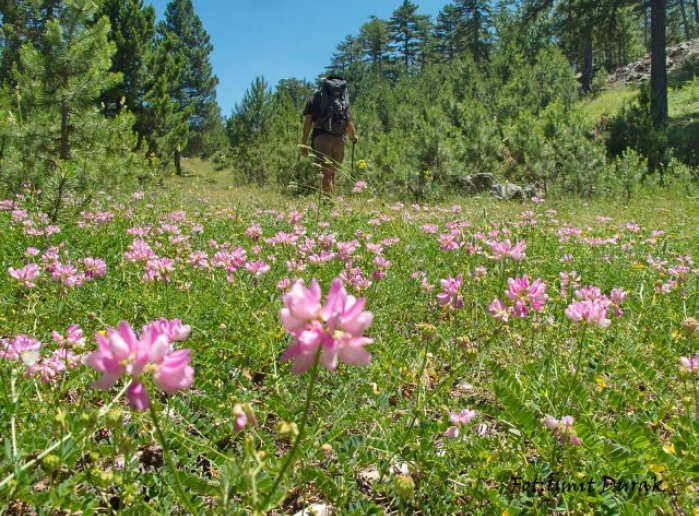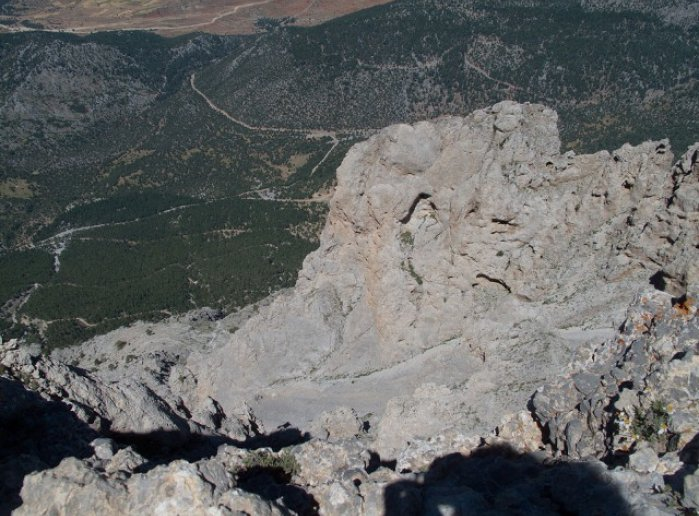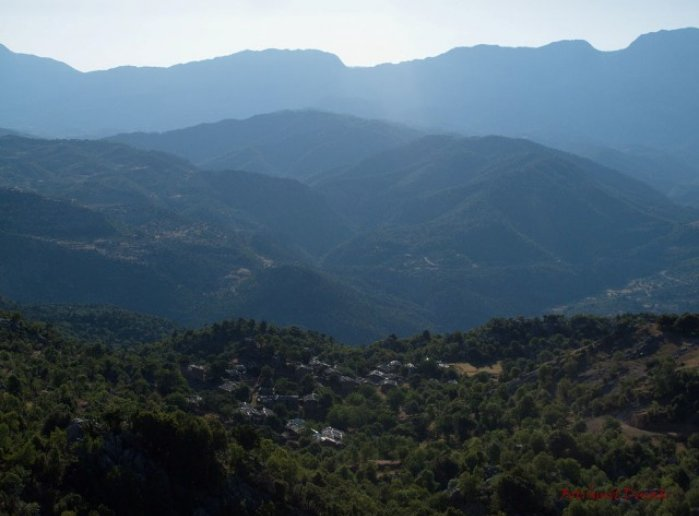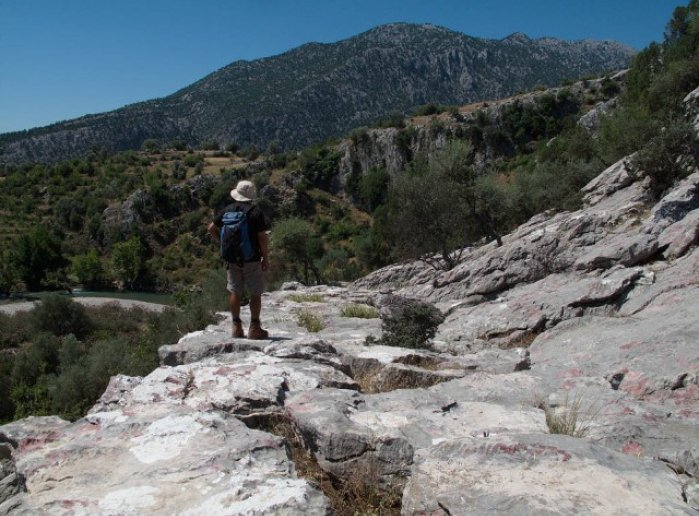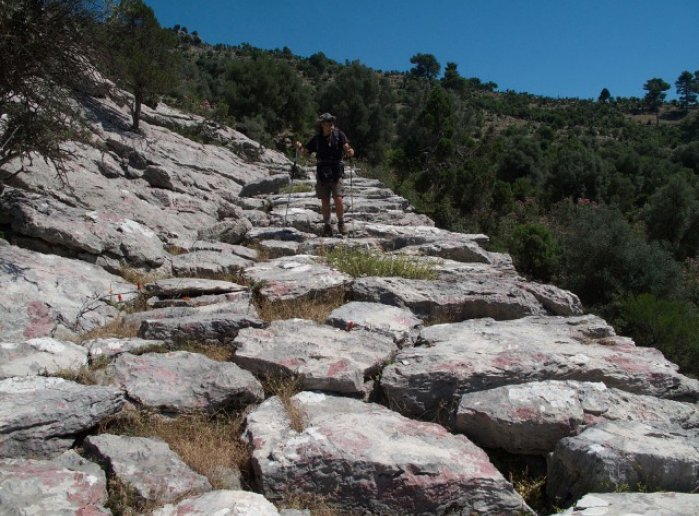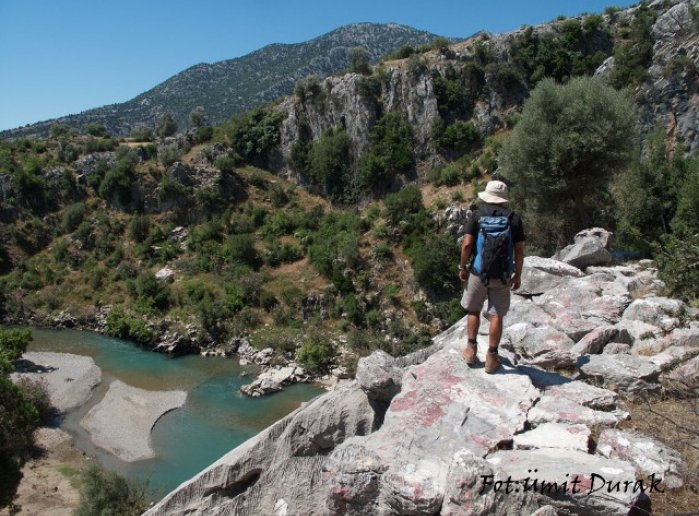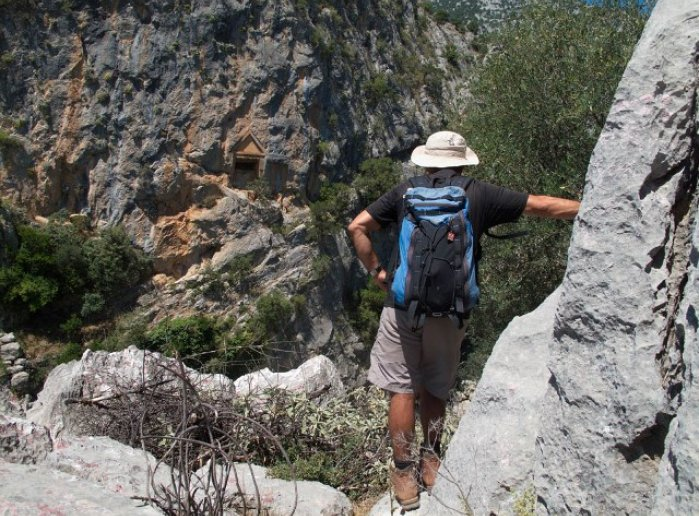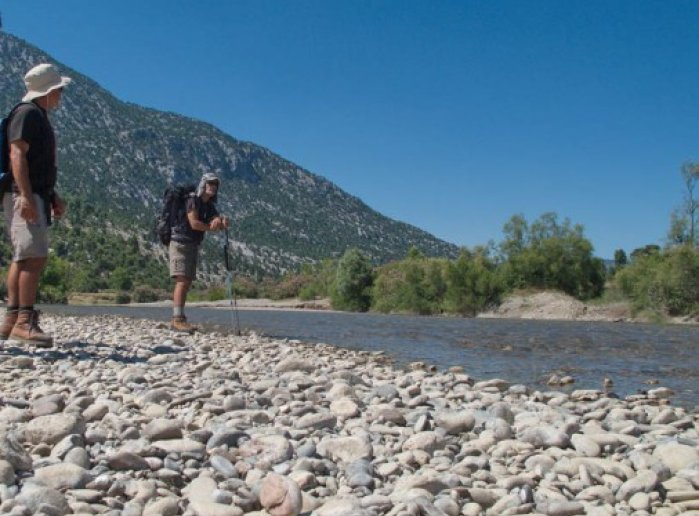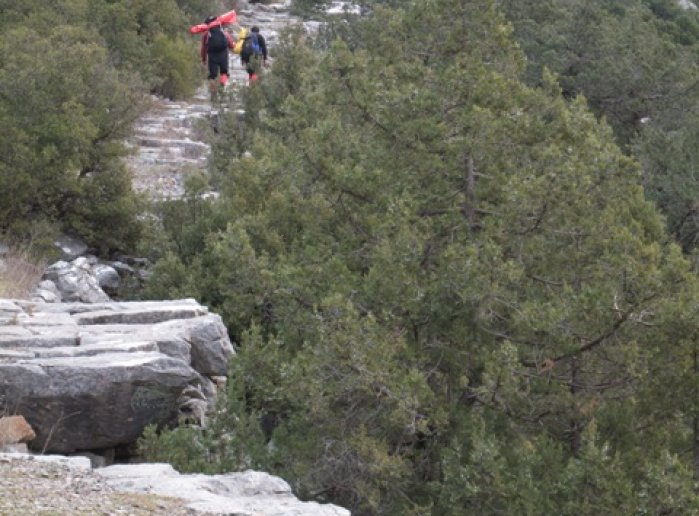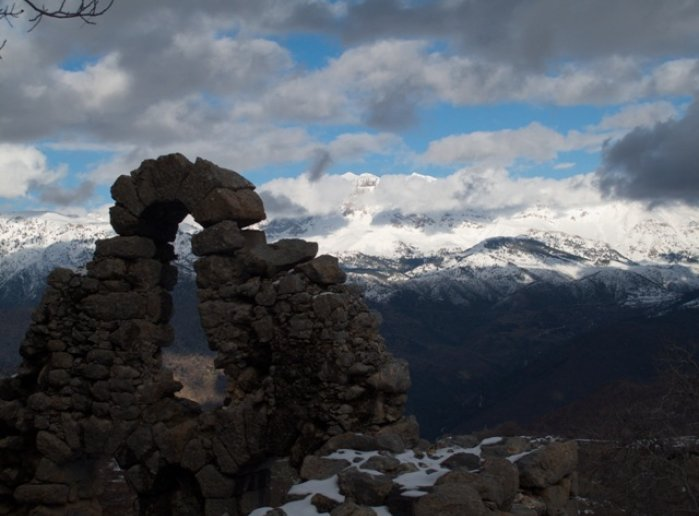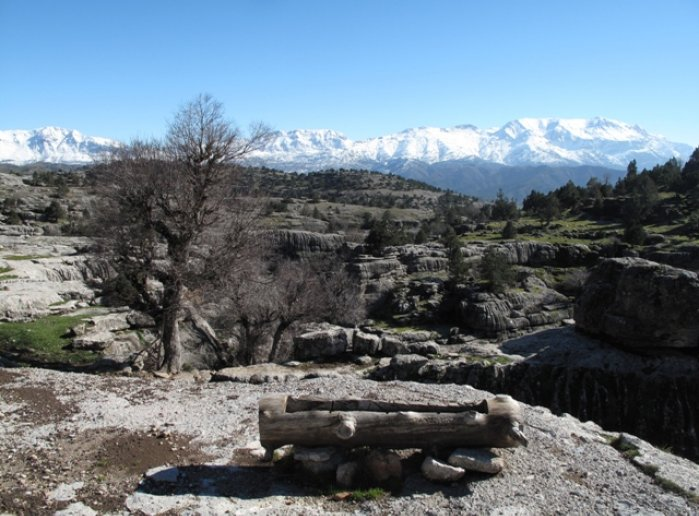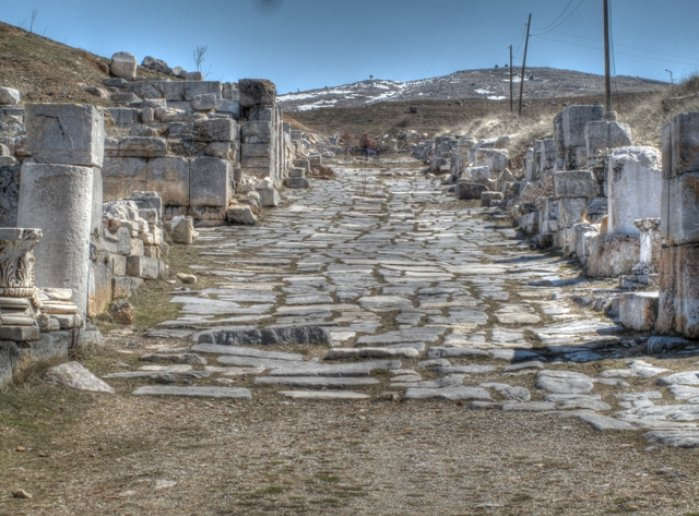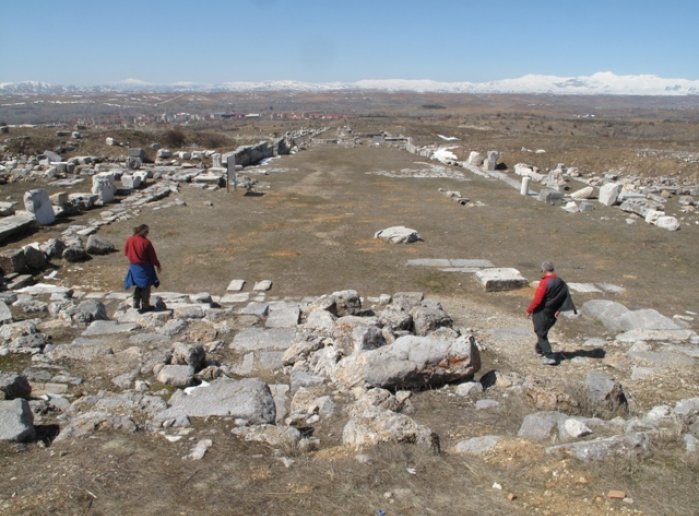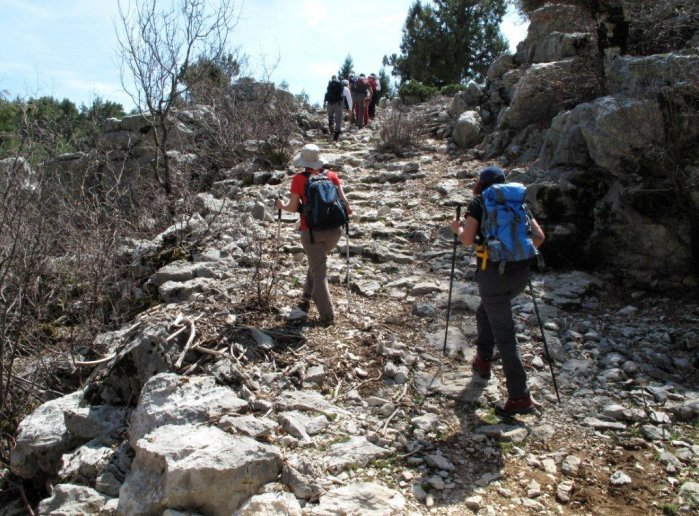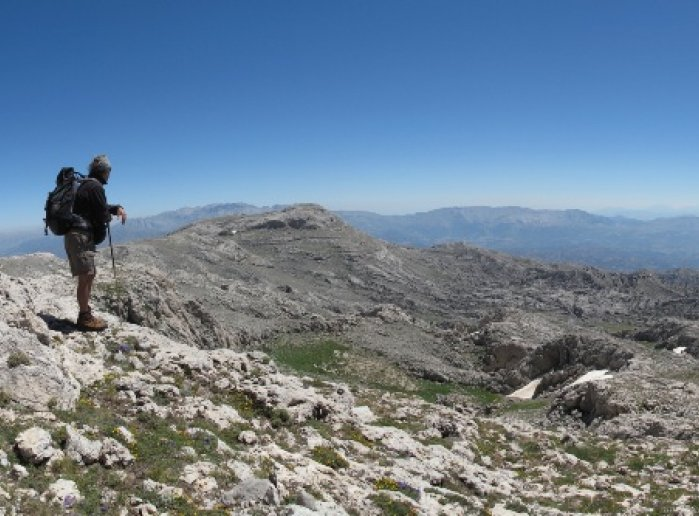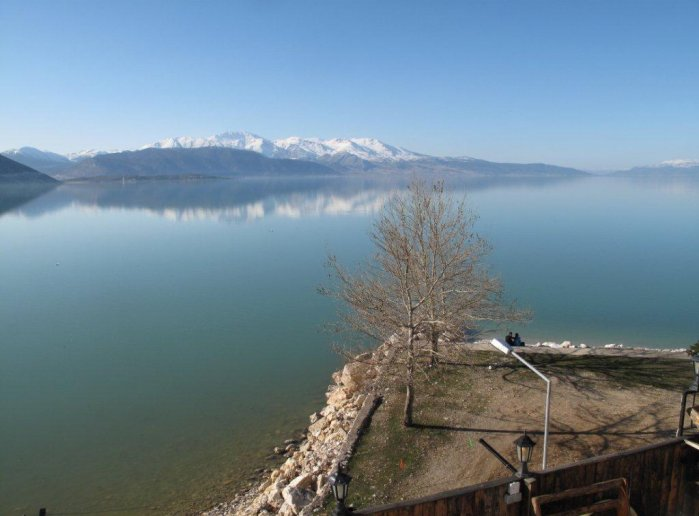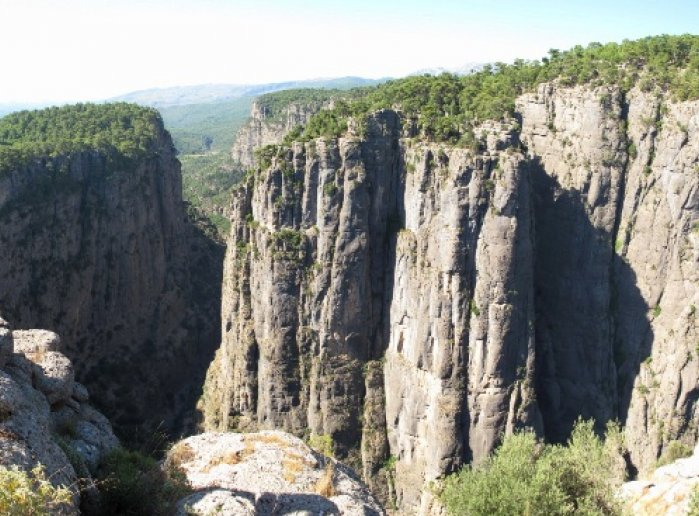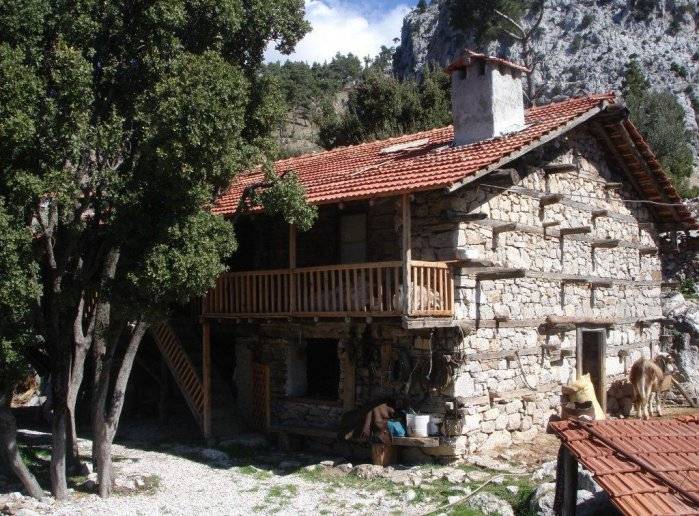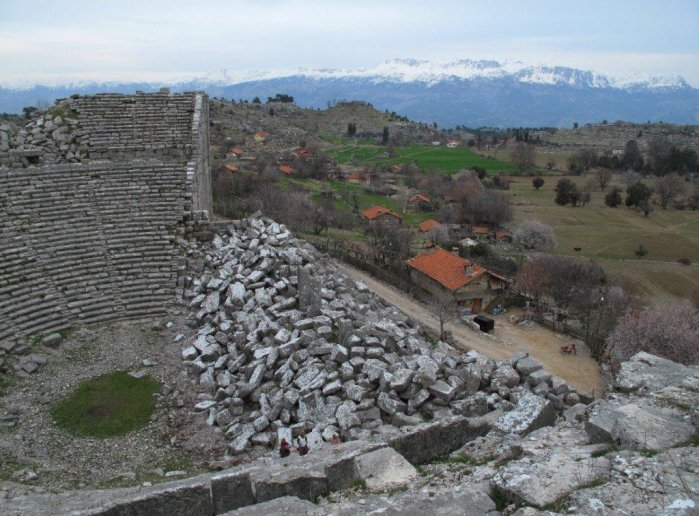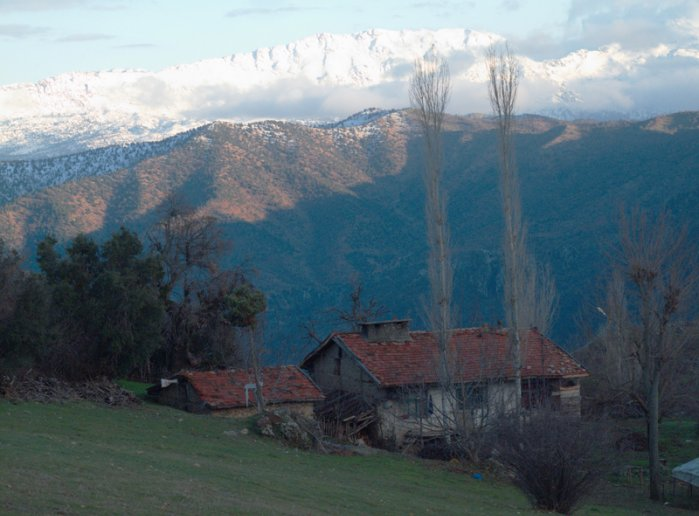Guided
ST PAUL WAY
Tour-Nr:
stp15
Duration:
10 Days
Persons:
4
Difficulty:
B
Location:
Isparta
899,00 €